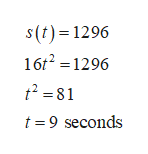s(t)=16t^2.Assume the distance from the top of the cliff to the water below is1296 ft. A rock is dropped off the edge of a cliff and its distance s​ (in feet) from the top of the cliff after t seconds isWhen will the rock strike the​ water?

Question
s(t)=16t^2.
Assume the distance from the top of the cliff to the water below is
1296 ft. A rock is dropped off the edge of a cliff and its distance s​ (in feet) from the top of the cliff after t seconds is
When will the rock strike the​ water?
Step 1

Given:

The distance from the top of the cliff after t seconds, when a rock is dropped off the edge of a cliff is s(t) = 16t2.

Step 2

Assume the distance from the top of the cliff to the water below is 1296 ft.

Therefore, se...help_outlineImage Transcriptioncloses(t) 1296 16t2 1296 = 81 2 t 9 seconds fullscreen

Want to see the full answer?

See Solution

Want to see this answer and more?

Our solutions are written by experts, many with advanced degrees, and available 24/7

See Solution
Tagged in

Calculus